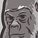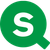# New to Qlik Sense

If you’re new to Qlik Sense, start with this Discussion Board and get up-to-speed quickly.

cancel
Showing results for
Did you mean:Contributor

## How to write set analysis for display current year

Hi,

I am using Qlik Sense and want to use set analysis to display Current Year and Previous year. I already try a few expression but not working to me. Can anyone help me ?

I have field name Order Date and sales in this case

Here is a few expression that I try :

`SUM({\$<Year={\$(=MAX(Year))}>}Sales)`

`SUM(\${<Year={"\$(=Year(Today()))"}>}Sales)`

Thank You

Nurul

2 RepliesMVP

How are you creating Year field in the script? Like this

Year(OrderDate) as Year? If it is like this... then this looks right to me

SUM({\$<Year={\$(=MAX(Year))}>}Sales)

or

SUM({\$<Year={\$(=Year(Today()))}>}Sales)Creator

You can use:

```Year(today()) // show the current year
Year(today())-1 // show the previous year based on current year
```

In yours expressions:

````SUM({\$<Year={'\$(=Year(Today()))'}>} Sales)   // sales for current Year`
`SUM({\$<Year={'\$(=Year(Today())-1)'}>} Sales) // sales for previous Year`
```Tags
Community Browser